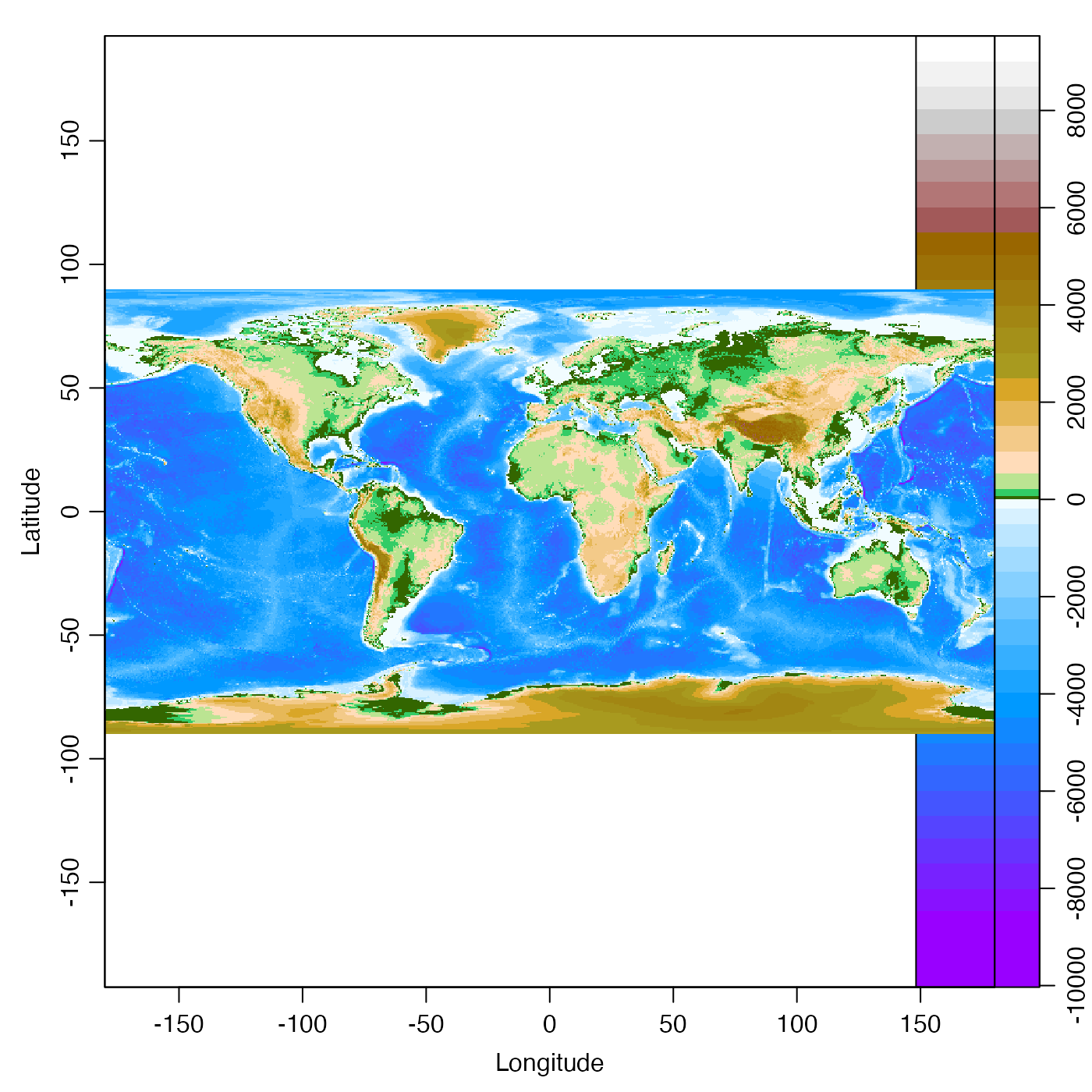Plot an image with a color palette, in a way that does not conflict with par("mfrow") or layout(). To plot just a palette, e.g. to get an x-y plot with points colored according to a palette, use drawPalette() and then draw the main diagram.

imagep(
x,
y,
z,
xlim,
ylim,
zlim,
zclip = FALSE,
flipy = FALSE,
xlab = "",
ylab = "",
zlab = "",
zlabPosition = c("top", "side"),
las.palette = 0,
decimate = TRUE,
quiet = FALSE,
breaks,
col,
colormap,
labels = NULL,
at = NULL,
drawContours = FALSE,
drawPalette = TRUE,
drawTriangles = FALSE,
tformat,
drawTimeRange = getOption("oceDrawTimeRange"),
filledContour = FALSE,
missingColor = NULL,
useRaster,
mgp = getOption("oceMgp"),
mar,
mai.palette,
xaxs = "i",
yaxs = "i",
asp = NA,
cex = par("cex"),
cex.axis = cex,
cex.lab = cex,
cex.main = cex,
axes = TRUE,
main = "",
axisPalette,
debug = getOption("oceDebug"),
...
)

## Arguments

x, y

These have different meanings in different modes of operation.

Mode 1. One mode has them meaning the locations of coordinates along which values matrix z are defined. In this case, both x and y must be supplied and, within each, the values must be finite and distinct; if values are out of order, they (and z) will be transformed to put them in order. ordered in a matching way).

Mode 2. If z is provided but not x and y, then the latter are constructed to indicate the indices of the matrix, in contrast to the range of 0 to 1, as is the case for image().

Mode 3. If x is a list, its components x$x and x$y are used for x and y, respectively. If the list has component z this is used for z. (NOTE: these arguments are meant to mimic those of image(), which explains the same description here.) Mode 4. There are also some special cases, e.g. if x is a topographic object such as can be created with read.topo() or as.topo(), then longitude and latitude are used for axes, and topographic height is drawn.

z

A matrix containing the values to be plotted (NAs are allowed). Note that x can be used instead of z for convenience. (NOTE: these arguments are meant to mimic those of image(), which explains the same description here.)

xlim, ylim

Limits on x and y axes.

zlim

If missing, the z scale is determined by the range of the data. If provided, zlim may take several forms. First, it may be a pair of numbers that specify the limits for the color scale. Second, it could be the string "histogram", to yield a flattened histogram (i.e. to increase contrast). Third, it could be the string "symmetric", to yield limits that are symmetric about zero, which can be helpful in drawing velocity fields, for which a zero value has a particular meaning (in which case, a good color scheme might be col=oceColorsTwo).

zclip

Logical, indicating whether to clip the colors to those corresponding to zlim. This only works if zlim is provided. Clipped regions will be colored with missingColor. Thus, clipping an image is somewhat analogous to clipping in an xy plot, with clipped data being ignored, which in an image means to be be colored with missingColor.

flipy

Logical, with TRUE indicating that the graph should have the y axis reversed, i.e. with smaller values at the bottom of the page. (Historical note: until 2019 March 26, the meaning of flipy was different; it meant to reverse the range of the y axis, so that if ylim were given as a reversed range, then setting flipy=TRUE would reverse the flip, yielding a conventional axis with smaller values at the bottom.)

xlab, ylab, zlab

Names for x axis, y axis, and the image values.

zlabPosition

String indicating where to put the label for the z axis, either at the top-right of the main image, or on the side, in the axis for the palette.

las.palette

Parameter controlling the orientation of labels on the image palette, passed as the las argument to drawPalette(). See the documentation for drawPalette() for details.

decimate

Controls whether the image will be decimated before plotting, in three possible cases.

1. If decimate=FALSE then every grid cell in the matrix will be represented by a pixel in the image.

2. If decimate=TRUE (the default), then decimation will be done in the horizontal or vertical direction (or both) if the length of the corresponding edge of the z matrix exceeds 800. (This also creates a warning message.) The decimation factor is computed as the integer just below the ratio of z dimension to 400. Thus, no decimation is done if the dimension is less than 800, but if the dimension s between 800 and 1199, only every second grid point is mapped to a pixel in the image.

3. If decimate is an integer, then that z is subsampled at seq.int(1L, dim(z), by=decimate) (as is x), and the same is done for the y direction.

4. If decimate is a vector of two integers, the first is used for the first index of z, and the second is used for the second index.

quiet

logical value indicating whether to silence warnings that might occur if the image is being decimated.

breaks

The z values for breaks in the color scheme. If this is of length 1, the value indicates the desired number of breaks, which is supplied to pretty(), in determining clean break points. If colormap is provided, it takes precedence over breaks and col.

col

Either a vector of colors corresponding to the breaks, of length 1 plus the number of breaks, or a function specifying colors. If col is not provided, and if colormap is also not provided, then col defaults to oceColorsViridis(). If colormap is provided, it takes precedence over breaks and col.

colormap

A color map as created by colormap(). If provided, then colormap$breaks and colormap$col take precedence over the present arguments breaks and col. (All of the other contents of colormap are ignored, though.) If colormap is provided, it takes precedence over breaks and col.

labels

Optional vector of labels for ticks on palette axis (must correspond with at).

at

Optional vector of positions for the labels.

drawContours

Logical value indicating whether to draw contours on the image, and palette, at the color breaks. Images with a great deal of high-wavenumber variation look poor with contours.

drawPalette

Indication of the type of palette to draw, if any. If drawPalette=TRUE, a palette is drawn at the right-hand side of the main image. If drawPalette=FALSE, no palette is drawn, and the right-hand side of the plot has a thin margin. If drawPalette="space", then no palette is drawn, but space is put in the right-hand margin to occupy the region in which the palette would have been drawn. This last form is useful for producing stacked plots with uniform left and right margins, but with palettes on only some of the images.

drawTriangles

Logical value indicating whether to draw triangles on the top and bottom of the palette. This is passed to drawPalette().

tformat

Optional argument passed to oce.plot.ts(), for plot types that call that function. (See strptime() for the format used.)

drawTimeRange

Logical, only used if the x axis is a time. If TRUE, then an indication of the time range of the data (not the axis) is indicated at the top-left margin of the graph. This is useful because the labels on time axes only indicate hours if the range is less than a day, etc.

filledContour

Boolean value indicating whether to use filled contours to plot the image.

missingColor

A color to be used to indicate missing data, or NULL for transparent (to see this, try setting par("bg")<-"red").

useRaster

A logical value passed to image(), in cases where filledContour is FALSE. Setting useRaster=TRUE can alleviate some anti-aliasing effects on some plot devices; see the documentation for image().

mgp

A 3-element numerical vector to use for par(mgp), and also for par(mar), computed from this. The default is tighter than the R default, in order to use more space for the data and less for the axes.

mar

A 4-element Value to be used with par("mar"). If not given, a reasonable value is calculated based on whether xlab and ylab are empty strings.

mai.palette

Palette margin corrections (in inches), added to the mai value used for the palette. Use with care.

xaxs

Character indicating whether image should extend to edge of x axis (with value "i") or not; see par("xaxs").

yaxs

As xaxs but for y axis.

asp

Aspect ratio of the plot, as for plot.default(). If x inherits from topo and asp=NA (the default) then asp is redefined to be the reciprocal of the mean latitude in x, as a way to reduce geographical distortion. Otherwise, if asp is not NA, then it is used directly.

cex

numeric character expansion factor, used for cex.axis, cex.lab and cex.main, if those values are not supplied.

cex.axis, cex.lab, cex.main

numeric character expansion factors for axis numbers, axis names and plot titles; see par().

axes

Logical, set TRUE to get axes on the main image.

main

Title for plot.

axisPalette

Optional replacement function for axis(), passed to drawPalette().

Logical value indicating whether to add to an existing plot. The default value, FALSE indicates that a new plot is to be created. However, if add is TRUE, the idea is to add an image (but not its palette or its axes) to an existing plot. Clearly, then, arguments such xlim are to be ignored. Indeed, if add=TRUE, the only arguments examined are x (which must be a vector; the mode of providing a matrix or oce object does not work), y, z, decimate, plus either colormap or both breaks and col.

debug

A flag that turns on debugging. Set to 1 to get a moderate amount of debugging information, or to 2 to get more.

...

Optional arguments passed to plotting functions.

## Value

A list is silently returned, containing xat and yat, values that can be used by oce.grid() to add a grid to the plot.

## Details

By default, creates an image with a color palette to the right. The effect is similar to filled.contour() except that with imagep it is possible to set the layout() outside the function, which enables the creation of plots with many image-palette panels. Note that the contour lines may not coincide with the color transitions, in the case of coarse images.

Note that this does not use layout() or any of the other screen splitting methods. It simply manipulates margins, and draws two plots together. This lets users employ their favourite layout schemes.

NOTE: imagep is an analogue of image(), and from that it borrows a the convention that the number of rows in the matrix corresponds to to x axis, not the y axis. (Actually, image() permits the length of x to match either nrow(z) or 1+nrow(z), but here only the first is permitted.)

This uses drawPalette(), and is used by plot,adp-method(), plot,landsat-method(), and other image-generating functions.

## Author

Dan Kelley and Clark Richards

## Examples

library(oce)

# 1. simplest use
imagep(volcano)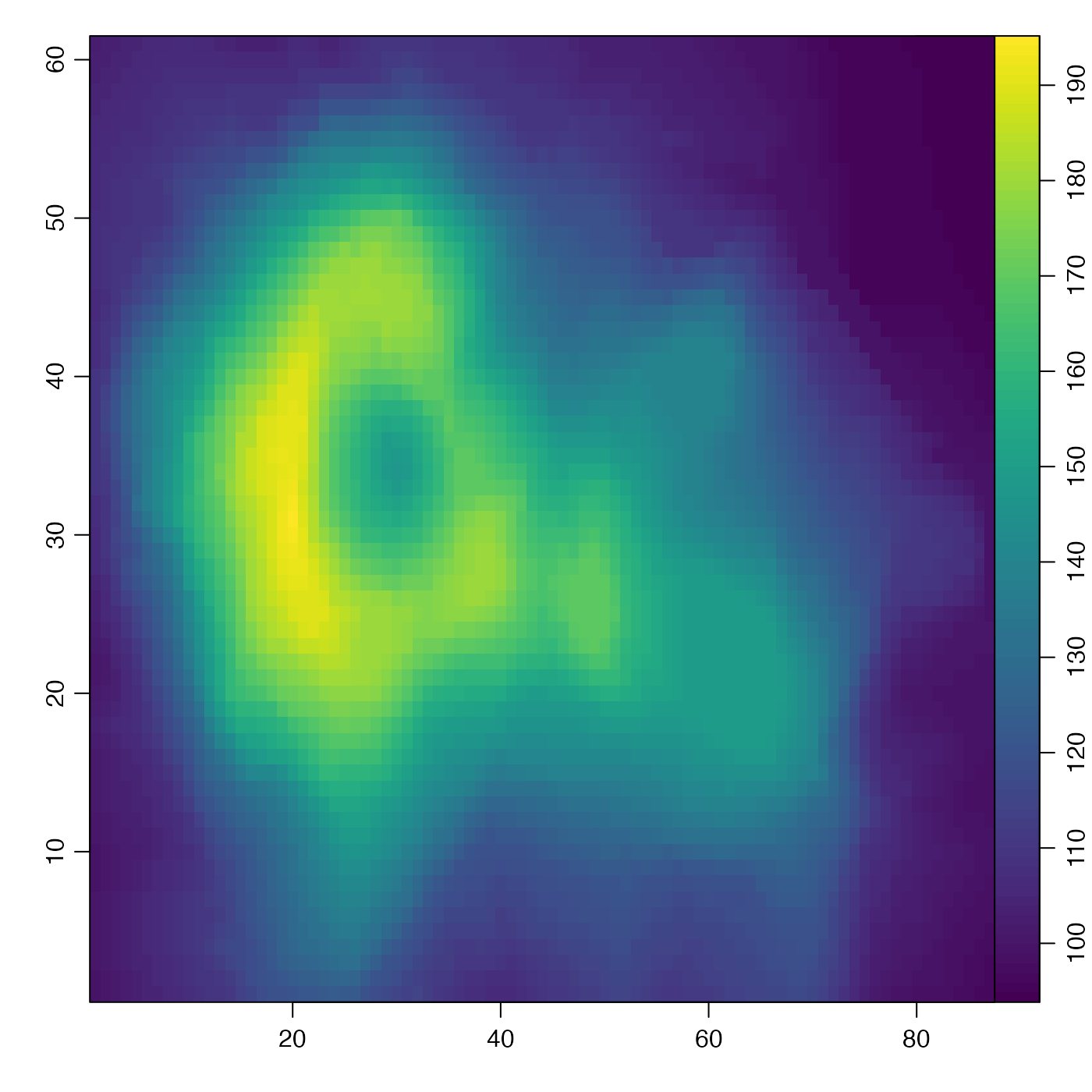# 2. something oceanographic (internal-wave speed)
h <- seq(0, 50, length.out=100)
drho <- seq(1, 3, length.out=200)
speed <- outer(h, drho, function(drho, h) sqrt(9.8 * drho * h / 1024))
imagep(h, drho, speed, xlab="Equivalent depth [m]",
ylab=expression(paste(Delta*rho, " [kg/m^3]")),
zlab="Internal-wave speed [m/s]")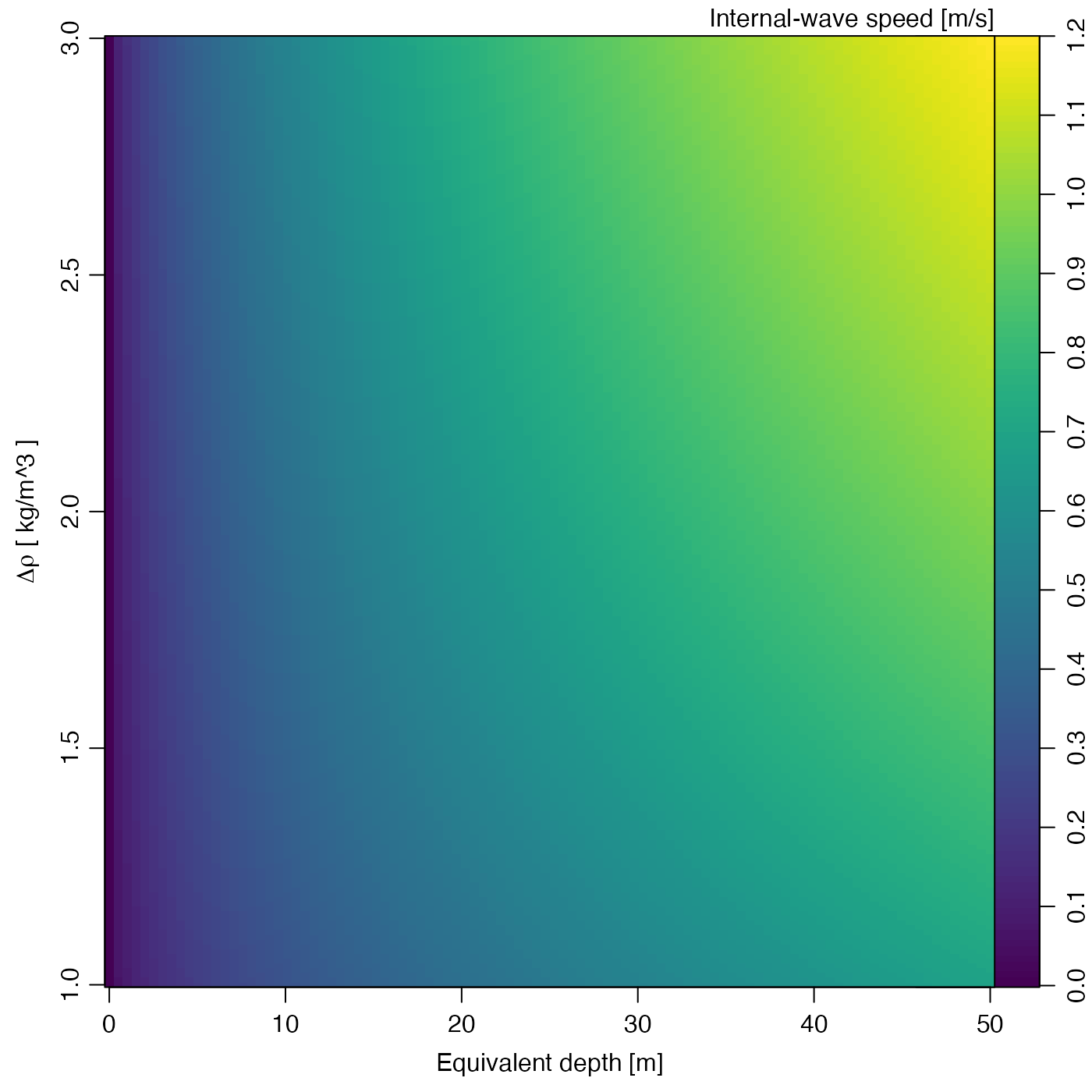# 3. fancy labelling on atan() function
x <- seq(0, 1, 0.01)
y <- seq(0, 1, 0.01)
angle <- outer(x,y,function(x,y) atan2(y,x))
imagep(x, y, angle, filledContour=TRUE, breaks=c(0, pi/4, pi/2),
col=c("lightgray", "darkgray"),
at=c(0, pi/4, pi/2),
labels=c(0, expression(pi/4), expression(pi/2)))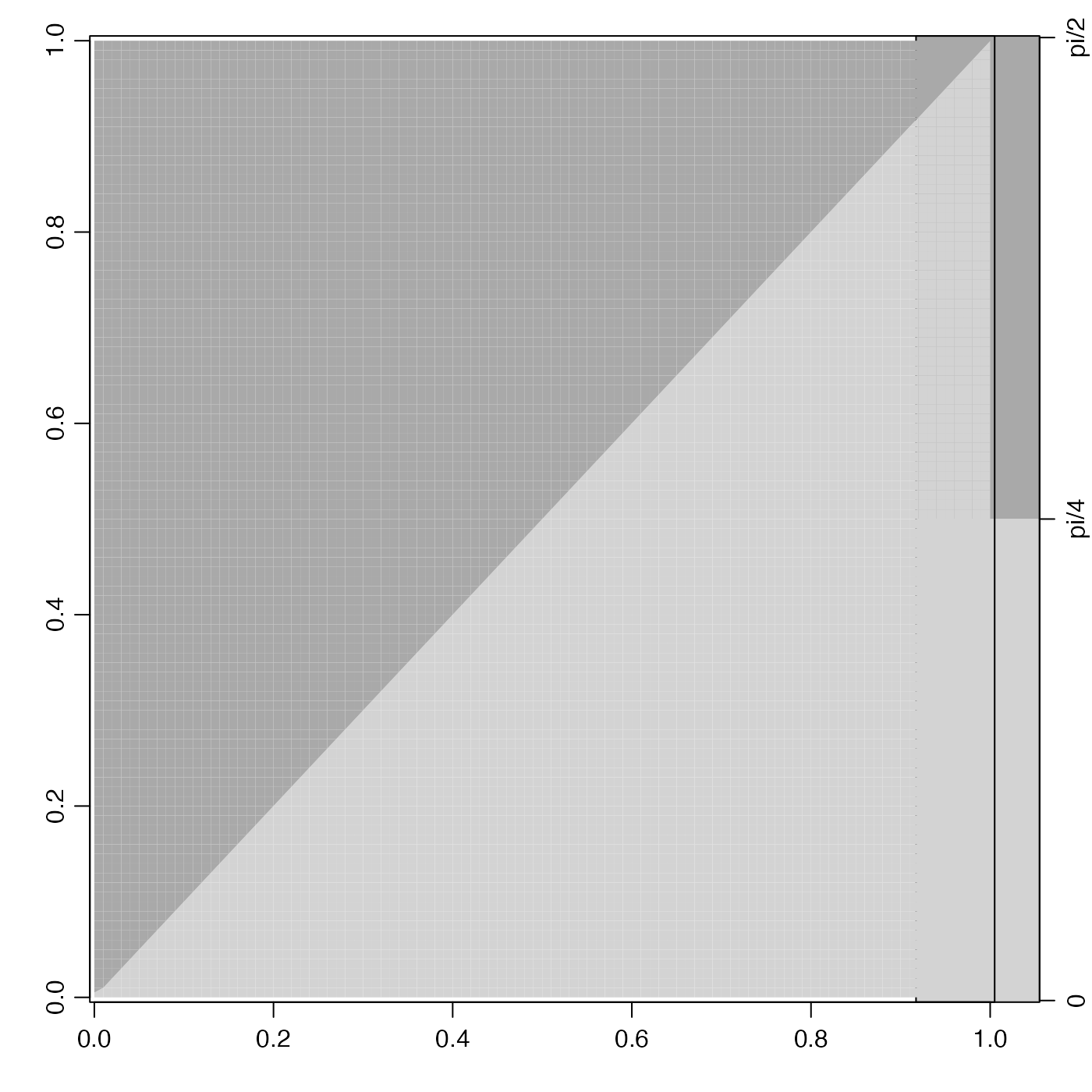# 5. y-axis flipping
par(mfrow=c(2,2))
imagep(t, d, u, drawTimeRange=FALSE)
mtext("normal")
imagep(t, d, u, flipy=TRUE, drawTimeRange=FALSE)
mtext("flipy")
imagep(t, d, u, ylim=rev(range(d)), drawTimeRange=FALSE)
mtext("ylim")
imagep(t, d, u, ylim=rev(range(d)), flipy=TRUE, drawTimeRange=FALSE)
mtext("flipy and ylim")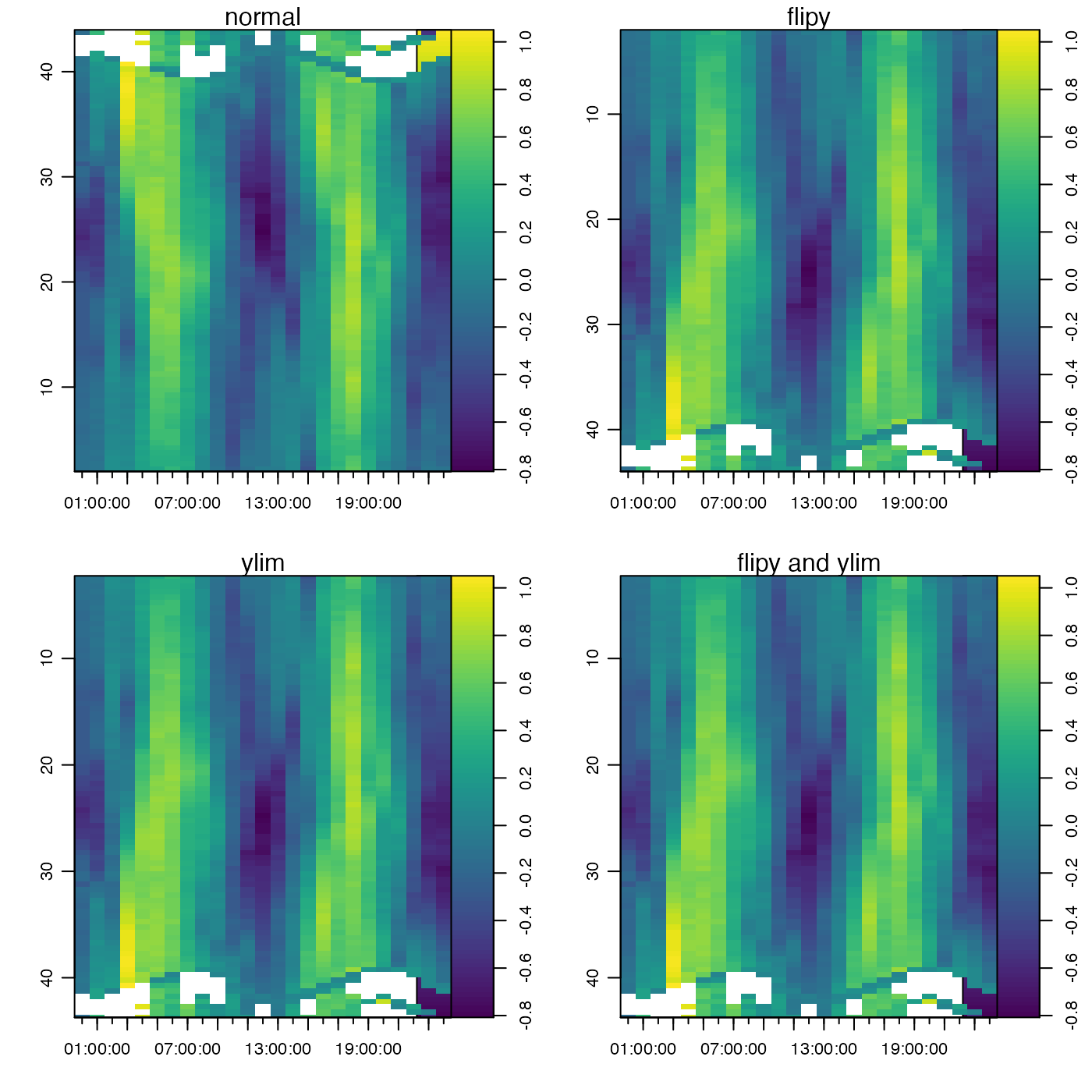par(mfrow=c(1,1))

# 6. a colormap case
data(topoWorld)
cm <- colormap(name="gmt_globe")
imagep(topoWorld, colormap=cm)